﻿ Role of Observer & Consciousness on Special Theory of Relativity and Its Influence on Kinetic EnergyPublications are Open
Access in this journal
Article Versions
Export Article
• Normal Style
• MLA Style
• APA Style
• Chicago Style
Research Article
Open Access Peer-reviewed

### Role of Observer & Consciousness on Special Theory of Relativity and Its Influence on Kinetic Energy

International Journal of Physics. 2017, 5(4), 99-109. DOI: 10.12691/ijp-5-4-1
Published online: June 15, 2017

### Abstract

Some of the basic concepts of ‘Special Theory of Relativity’ reviewed once again and emphasized the concept of absolute velocities in this nature. Physical interpretation of these transformations introduced the ‘principle of absolute velocities’ and its relation with observed velocities has been explained. Films of the ‘Film theory of the Universe’ have been explained as ‘Inertial frames of Reference’ of ‘Special Relativity’ and emphasized the concept of ‘consciousness’ in physics. The conclusions suggested that the particle with a mass subjected to kinetic energy can be calculated by both ‘special relativity’ and ‘modified concept of relativity’ i.e ‘concept of absolute velocities’. These two are producing different results. These results can be verified in any ‘high energy laboratory’. Thus it claims for an experimental verification for kinetic energy in support of modification in ‘Special Theory of Relativity’. The observed velocity of ‘concept of absolute velocities’ should be considered for all mathematical calculations instead of relative velocity. It is concluded that the ‘Special Relativity’ is applicable for a single film of the Universe. Life or consciousness of living things must have at least two films and transformation between films generate the sensation of existence of the materialistic or physical universe. Thus this concept is very much useful for ‘physics of consciousnesses’.

### 1. Introduction

This is an original thought on basics originated from the following questions.

Why light velocity is constant for all inertial frames of reference?

If accelerated frames explained by special relativity 1 requires a momentary inertial frame 2 for explanation, then what is that moment at which the accelerated frame behaves as an inertial frame and will be carried out to next inertial frame within a moment of time? What is that moment of time?

Why does the twin paradox using the word ‘rest frame’ 3 if velocities are relative? Is the velocity of twins are not the same when one is moving with another? Why one is moving frame and another is rest frame? Special Relativity started from transformations from one inertial frame to another. So time dilation occurs for a relative velocity. Now if we think reversely i.e time dilation represents the flow of time or run of a clock, the inertial frame can be defined as the points in space contains equal time dilation. This can be called as an inertial frame with a particular velocity and another frame which will have different time dilation will be another inertial frame of reference. If this is the case ,we can remove the concepts ‘rest frame’ and ‘moving frame’. Then analysis and results will be different for twin paradox. Why don’t we think in this way?

These basic questions lead to look in to a new dimension of inertial frames of reference and the transformation between them. It also emphasizes the importance of observer and observation. The most basic transformation must consider the involvement of ‘consciousnesses’ in the field of physics. Here consciousness has been taken as equivalent to life. Means, all the living things will have consciousness and that is the main constituent that shows the difference between living and non living things. The degree may vary and defined differently in different fields like medicine, psychology, biology etc.

This outlook substitutes the relative velocities concept with ‘absolute velocities’ and modifies theory of relativity accordingly. The modified theory gives a different equation for kinetic energy of a particle which is different from the equation of kinetic energy derived by special theory of relativity. The results tabulated and can be verified for any particle with mass ‘m’ and velocity ‘v’. This prediction proves the concept of absolute velocities. This concept of absolute velocities is use full in dark matter calculations, origin of universe theories, black holes and physics of consciousness. The conclusions emphasized the importance of this ‘theory of absolute velocities’ in physics of consciousness.

### 2. Discussion

The most basic of relativity is ‘all the velocities in this nature are relative to each other except light. If we take two object moving with a relative velocity ‘v’ to each other, as per relativity, the time dilation, length contraction and mass change must be same in both the frames. There is no meaning in saying that one is rest frame and another is moving frame. Both the frames are moving each other so the changes must be same in both the cases.

If we take example of ‘twin paradox’ 3, one twin will go in to space and returns back in certain time. Then the twin on earth will be more than the others age. Here the question is – if the twin left the earth moves with a velocity relative to the twin on earth, the twin on earth which is a zero velocity relative to earth will also move relative to him. So the change in time is same in both. Then how can we say one is rest frame and other is moving?

Even if he travel and come back, his clock will be less than clock on earth and clock on other twin. Means, he will be younger than the other twin. We can agree for it. But for him, after coming back to earth, the events are same. That means the events are happening faster on earth like biological changes such as age and death of people on earth will be faster relative to the twin who is in journey. But clocks will show same for both the twins. He has changed so many frames. In each frame his clock was changing. Finally he came to earth and the total time will be reduced relative to the twin on earth because of time dilation due to special theory of relativity.

Here he has jumped lot of frames. The other one on earth has no change. So other one is rest frame and the jumped is not in rest. Also the jumping of one frame to another is nothing but change of velocity with time and can be said as non inertial frame i.e accelerated frame. Is it correct?

The jumped frames are all inertial frames only. Or there exist a momentary inertial frame equivalent inertial frame at that moment when a signal is released from that accelerated frame. So the physical transformation is connected to each inertial frame of twin moving in space and the frame of the twin on earth. So the changes should be same. So whatever time change is there, that will be applicable to both the twins. So there will not be age difference even after journey. But for him the twin on earth will not die and people will be young only. But for other people on earth, people including twin will become old and die.

It is not at all possible (dead and alive is not possible at the same time).

2.1. Thought experiment& Analysis

Figure 1 shows that observer A is on earth. B&C are twins. B started to travel in to space. Fist B is also zero velocity and then reached to a velocity ‘v’ . This velocity ‘v’ is relative to C . Velocity of the C is zero with respect to A. Now for B, the velocities of C and A are also with velocity ‘v’. Let us consider B and C , B is with velocity ‘v’ relative to C and we have to accept that the velocity of C is also ‘v’ with respect to B . Now Let us consider four positions of the space craft which has left earth and will come back to earth after some time and the velocity is just near to velocity of light.

Suppose the space ship reaches position ‘2’ is at a distance from earth is ‘4 light years’ and the velocity is 0.8c.and returns with the same velocity to earth i.e position 4.

Then the round trip will take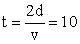years as per earth’s time (on earth 10 years will be passed for all). But the twin moving in space ship will get time dilation due to its velocity. So for him the same 10 years will be affected by relativistic factor. The relativistic factor is ‘ ’ and is equal to 1/0.6 then the 10 years time of earth will be 10/(1/0.6) = 6 years for the twin who is travelling. Thus when they meet after journey of one twin, the twin on earth will have 10 years of age and the twin who travelled will be of 6 years old. This is the explanation given by special theory of relativity.

We cannot deny the calculations based on special relativity. But fundamentally we can raise two questions in this twin paradox.

1. What is rest frame? Why one of the twin is in rest frame another is in moving frame when velocity is relative to both. When velocity is relative, the changes must be same for both the twins.

2. The velocity is not uniform velocity. It is changing from 0 to v, v to 0, in one direction and 0 to v ,v to 0 in return direction and finally reaches the other twin. This change of velocity denotes acceleration. Can this acceleration be explained in terms of inertial frames of reference?

3. As per the present view, accelerated frames can be explained by special theory of relativity as a virtual or momentary inertial frame relative to accelerated frame 2. So many relativists expressed their views. For example, an internet article entitled physics FAQ originally by Philip Gibbs 1996 ‘Can special relativity Handle acceleration? Stated that “acceleration measured in ‘momentary commoving inertial frame’ is always the same’; this frame is an inertial frame travelling at the same instantaneous velocity as the object at any moment” 2.

2.2. A Different View of the Above Analysis

In relativity we cannot say one is moving and another is at rest. If one moves with velocity relative to other it will be vice versa also. Both the objects will be relative to each other. For accelerated frames we cannot imagine an momentary or instantaneous inertial frames of reference since zero to infinite momentary or instantaneous frames will exist and the duration of this moment is zero to infinite. It cannot be acceptable for quantum concept of time. So the following explanation is given for twin paradox and the thought experiment concludes another different version of twin paradox which is different from the version of special theory of relativity. By this, a new concept of ‘absolute velocities’ originated and definition of Inertial frame of reference precisely stated. Here transformations from one ‘inertial frame of reference’ to another ‘inertial frame of reference’ described as acceleration.

Position.1: At this position, B will have velocity ‘v’. So as per relativity, time contraction will be there. Let us suppose this velocity is 0.8 times velocity of light. And the distance is 2 light years from C. So it takes 2.5 years to reach position 1. Here relativistic factor is 0.6 so the time will be 1.5 years after considering time contraction due to velocity. As per me, at this position both clocks at C and B will have same time dilation. That means the duration between two ticks of both the clocks will same. So if B has reached position 1 after 2.5 years, for A the time 2.5years will be 1.5years for B. Thus the time is reduced.

Position 2: At this position velocity of ‘B’ is zero (since it has to return back).In this position also the time dilation of B& C will be same. Means both the clocks will run similarly (the duration between two ticks will be same).

Position 3: At this position again ‘B’ will get velocity ‘v’ and clocks for both the B and C will run similarly. But relative to position 2 its clock will run slowly. Now it’s velocity will be reduced to zero and reach position 4.i.e. initial position.

Position 4: It is the initial position from where one of the twin i.e ‘B’ travelled to positions 1,2,3 and back to initial position. In this position also the clocks will move similarly (two tics of clocks at B and C will be same. But the clocks will move faster than the previous position i.e. position 3

2.3. Observations in the Analysis

At all positions the clock in B and the clock in C will be same means, time dilation will be same and the flow of time or running of clock will be same. There will not be any change in their clocks relative to each other at that particular position.

But relative to A, C is at rest and B is moving. So B will experience time dilation since it is moving with velocity ‘v’. If the travel time for B with respect to A and C is 10 years , in addition to travel time it will experience time dilation also so the clock for B will be slow than C if they meet after the journey.

Let us suppose the travel time for B is 100 years with respect to A and C. Suppose both B and C are twins and for them all the biological changes will occur at same ages if both are rest to each other (For example both B and C will start crawling at 2 years of age. Both will walk at age of 3 and both started talking at 5 years. Similarly both will get beard at 12 years, both will get gray hair at 40 and both will die at 70 years).

Now as per Einstein, because of travelling in space ship with some velocity comparable to light, the clock will get slow and suppose that the travelling time reduced due to time dilation by 5 years with respect to twin C . After 70 years if they planned to meet, the C will die at 70 and still B will have 65 years and alive.

But as per me, both will die. But C will die at the age of 70 and B will die at 65 years

2.4. The Reason

At all the positions 1, 2, 3 and 4 the clocks of both B&C are running similarly. Time dilation will be there at position 1 relative to initial position i.e position 4. So clock of B will be slow since relative velocity exist and at position 2 time dilation will not be there relative to initial position and flow of time (duration between two clicks) will be same. In position 3 again time dilation will be there and clock will be slow for B. Again at position 4 clocks will run similarly for B&C. Any how the events will be similar at any position relative to initial position (basic idea is- if B moves with velocity relative to C , C also will move relative to B). So at 2 years of age both B&C will crawl. Both will walk at age of 3 and both starts talking at 5 years. Similarly both will get beard at 12 years; both will get gray hair at 40 both will die at 70 years. But the age of B will be less than C since it has travelled. Finally when they meet at initial position, the time dilation in both are same (the duration of two ticks same) i.e flow of time is same for both B&C. After 70 years of age C will die and but for B it will be of 65 years. But as per the 2nd condition that the flow of time will be same in all positions, and events will be similar in all the positions relative to each other, the event ‘death’ will occur to both B&C in position 4 when they reach the initial position.

The difference in both the cases is because of relative velocity only.

1. Is rest frame and moving frame in relativity are correct?

2. What is momentary ‘‘inertial frame of reference’ for an ‘accelerated frame’?’ Can there exist any ‘inertial frame of reference’ at a particular moment of time to send a signal from moving frame to rest frame?

### 3. Theory of Absolute Velocities

It says that ‘inertial frame of reference’ is nothing but ‘the frame in which time flow is same’ means at all points in inertial frames of reference, the clocks are same and time dilation will be same and the duration between two ticks of clocks for all points in that frame is same. Finally we can say flow of time is constant.

In the above example at the positions1, B & C will contain a frame. Here, if we consider A also in that frame A, B&C will have a frame at position one. That will be different with the inertial frame of reference of A,B&C at position 2 and position 3 & 4 also. There will exist lot of positions. So lot of frames also will exist and are different from each other.

So ‘inertial frame of reference’ is defined by its flow of time i.e time dilation. That will be from zero to infinite. In other words we can say that an inertial frame will contain a velocity but it is not relative. It is absolute and for all other inertial frames of reference, its velocity constant. We cannot do any transformation from an inertial frame of reference to another. Special theory of relativity is valid for one particular frame only. But universe is made up of such lot of inertial frames. So lot of stationery frames will exist for an event

Let us see how to interact from one inertial frame to another?

Relativity introduced ‘accelerated frames’ to describe it. We cannot deny that. Here they have introduced involvement of ‘fictitious’ forces to describe the interaction. But how far is it relevant? If we go profound analysis, the interaction can be explained in the following way.

What is the meaning of momentary frame?

There are lot of frames with duration of two ticks of clocks from zero to infinite.

All these frames are absolute velocities with relative to each other means its velocity is constant for all other frames.

Observer will be in a frame with a zero velocity. The signal for interaction between any two frames is maximum velocity i.e infinite which is the frame of reference for light. In this frame signal will transfer from one point to another with infinite velocity since the travelling time is zero. In this, the duration between two ticks of clock is zero and time flow is infinite.

Now for transformation from one frame to another, we have to imagine equivalent frame of reference which is at rest with the absolute frame. Then the Figure 2 shows the relativity behind it and how the observation will be made by observer. This velocity has to be considered for all observations and can be called as observed velocity.

Here let us take the example of frame contains velocity of light

As per the description, the velocity of the object in that frame which is at light velocity will be observed as ‘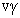’ ie infinite since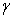is infinite when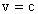. But its absolute velocity is c only.

Its absolute velocity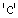means, its velocity is constant for all inertial frames of reference. Here the observed velocity is infinite since it is ‘’.

Thus if velocity of light is constant for all inertial frames of reference its velocity is more than that of light i.e infinite.

Thus Einstein’s first postulate contradicts the second postulate.

If both the postulates are right then only special theory of relativity will be correct hence special theory of relativity is not relevant.

Then what is the solution?

We can take the maximum observed velocity is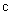.then if we apply the theory of absolute velocities, we can find out the absolute frame for observed velocity.

Therefore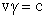Represents the maximum signal velocity as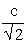.

Therefore, Relativistic factor for absolute velocity=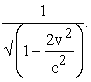This is absolute frame and it is maximum absolute frame. Now all the frames can be transformed by this frame and observed velocity can be found.

We have mentioned positions in the above analysis or thought experiment illustrated in Figure 2. What is position? How many positions will be there in between position 2 to 3 or 3 to 4. Infinite positions will exist since positional change may be at any duration of time dependent on the force applied. It will be from zero to infinity.

But zero time duration possible?

If the duration between two tics is zero means time flow is of infinite. Speed and velocity of signal is infinite. But velocity of light is constant. So the time duration between two clocks also should have a minimum limit. A fraction of second. Let us call it as quantum of time. Duration less than this time will not exist.

It is already explained in ‘film theory of the universe’ 4, 7, 8. Figure 3 shows the arrangement of films of the universe as per ‘film theory of the universe’.

Let us consider the analysis (section. 3) once again-

The above referred frames will follow principle of absolute velocities. Here principle of absolute velocity is “All the velocities in this universe are observed velocities and must be converted to absolute velocities for the purpose of transformations. Absolute velocity multiplied by relativistic factor is equal to its observed velocity”. So whatever velocity we are observing in the universe will be an equivalent absolute velocity and it will follow ‘principle of absolute velocities’. So its absolute velocity is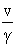and is constant for any point in this universe.

Here as per our observational facts, we know that the universe is expanding and obeying the Hubble’s equation ‘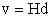’. Here if we assume the universe is existed withthen we can get a graph between ‘’and ‘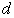’ this will show that the same expanding force i.ewill have another form as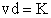. Where ‘K’ is siva’s constant 5, 6, 7. Thus these two will exist in absolute frame as expansion and contraction. Contraction is nothing but gravity. This is existed for a particular absolute velocity. So it is a frame in which both expansion and gravity exists. But it is not transferred to other frame. A mass in this frame when transferred to another next frame, we can find the time between the film and another next film. Now it is limited to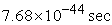4 dependent on the universal limit like light velocity.

This small infinitesimal portion of time after which the time cannot be divided further has been predicted by film theory of the universe. Say it is quanta of time i.e. ‘Double Relativity Effect’ 4 and ‘film theory of universe’ emphasizes that the universe exists in films and in this paper it is explained that film can be treated as a frame.

This quantum of time is very much required in this context because, the frame or film at position of universe with in thisof quantum of time represents space only. Not the space time. This obeys completely the definition of inertial frame of reference. Here acceleration will not be there since time will not be there. But velocity will be there and it is uniform velocity only. Its uniform velocity will be zero for one film and varies with film to film from zero to infinite since we have changed the definition of inertial frame of reference as- the frame connecting all the velocities with equal time dilation. Means, in one such frame the flow of time is constant and the clock will be same for all points of that film or frame. At this quantum of time, the time dilation will be infinite and there will not be flow of time.

Here if we think about accelerated frames, all the accelerated frames can be expressed as changing inertial frames with time only. Now the question is which particular position is to be considered to trigger the signal and obey the rules of special theory of Relativity? There is no answer for the question, if time can be made to infinite divisions. But time cannot be of infinite divisions. Its quantum isonly. So at this position signal can be transformed and special theory of relativity will be followed.

At this time of quanta i.e., the film of complete universe is in the form of space only. There will not be time. But time isfor all the points in that space. So it can be termed as an ‘Inertial Frame of Reference’ and it is eligible for application of ‘special theory of relativity’.

In the nextone more film will be existed. This is also ‘inertial frame of reference’. for the first film the time dilation isand next film is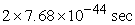. So time dilation is different for both the films.

These two are two separate ‘inertial frames of references’. So signal cannot be transferred from one to another. For this transformation, we should apply the concept of consciousness into physics.

Till now there is no precise definition for consciousness in science. There are various definitions in medical terminology, psychology etc., for consciousness. We have to freeze one definition for all the subjects. Then we have to think what life is? Why consciousness is attributed to living things only? What is the difference between life and consciousness? Then we have to define life and the difference between living things and non living things. Physics is describing universe and all materialistic worlds. It is very deterministic. Then why it is not describing the difference? It can explain deterministically. Physics of consciousness elaborated by prof. Max Tegmark can be referred in this context 9

First of all let us consider life itself is consciousness, of course degrees may vary 10

Next step we can do is in the scale of its order. We can say various levels of life. In that, consciousness also will be one of level can be described deterministically and perfectly.

The definition given by physics which starts from a concept and to reach direct or indirect verification by experiments will satisfy all the subjects like medicine, psychology, physiology etc. Thus the attempt to describe the difference between living and non living things by physics has been initiated by super theory of relativity 4.

As per super theory of relativity two consecutive films are connected by consciousness.

Here the space time arrangement is alternative as shown in Figure 4. In it every single film is of space and then converts completely in to time. Again it will convert in to space. Thus alternatively space and time will exist. So the combination of space and time shown as ‘space-time’ will also exist alternatively. The time duration will be added to the next one and the next one and so on. So space time is also desecrated. But related by general relativity principle and as the time increases the space also increases. Thus different films or frames will exist.

And the transformations are as per Figure 2

The same if we consider once again, it is obvious that there will exist lot of ‘inertial frames of references’ with different time dilations. Means, there exist lot of velocities (absolute) since the observation is a link between one ‘inertial frame of reference’ to another represented by one ‘absolute velocity’. Since it is an absolute velocity, it is constant for any observer and can be called as observed velocity with application of ‘Double Relativity Effect’.

Here it is not objecting the concept of accelerated frames. In accelerated frames, mathematically, change of ‘inertial frame of reference’ or velocity is a function of time. Accelerated frames in special relativity explained by a momentary frame relative to the inertial frame 2. The same is mathematically explaining the general relativity with a fictitious force like gravity and explained the space time continuum and the curve as gravity.

But what is the meaning of ‘momentary’? Time cannot be divided as infinite as explained above. So ‘momentary’ is not reasonable. That means there will not exist any momentary reference frame between two inertial frames of reference to happen any event. Thus the present explanations are not reasonable. All inertial frames of reference or films of universe obey ‘special theory of relativity’. ‘Double Relativity Effect’ is required to connect one film to other.

Now we can say all the velocities are absolute and associate to any one of the film of the universe as per its time dilation. If it is an absolute velocity, its velocity is constant for all velocities from zero to infinite. Thus there exists zero to infinite inertial frames of reference in this universe. Observer will be always at zero velocity and signal will be always at velocity of light to cover all these inertial frames of reference.

Then if we apply ‘Double Relativity Effect’, the observed velocity is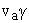. That is the velocity we are using in special relativity, accelerated frames, general relativity etc. The mathematics applied in these are not denied but the results cannot be with mathematical formulations, the results will vary since the physical existence is different.

So with the following postulates will lead to a ‘theory of absolute velocities’.

3.1. Postulates of the Theory

1. The points in space in which flow of time (clock) is same are called as ‘Inertial Frame of Reference’. Signal can be transferred from one point to another in it.

2. A film of the universe proposed in film theory of the universe is an ‘Inertial Frame of Reference’

3. A momentary frame cannot exist for an accelerated frame to send a signal for a transformation from accelerated frame to inertial frame.

This concept of absolute velocities will remove the problems of relative velocity concept.

Velocity of light is associated to an absolute frame with velocity of light and it is the maximum absolute velocity. All the velocities in this universe are observed velocities containing an absolute velocity associated to every observed velocity and follows ‘Double Relativity Effect’.

### 4. Derivation of Kinetic Energy as Per ‘theory of absolute velocities’

Let us suppose a particle of rest mass ‘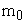’ is moving with a velocity relative to an observer.

Rest mass isand observed velocity is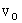. So the classical notation for Kinetic Energy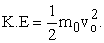(1)

As per special theory of Relativity the Relativistic equation for Kinetic energy is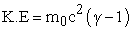(2)

Where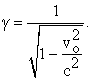As per ‘Double relativity Effect’, with reference to the Figure 2.

Special relativity will be applied and the observed rest mass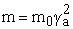(3)

Where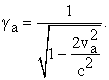And Observed velocity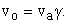(4)

Now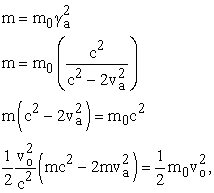Observed Kinetic Energy

i.e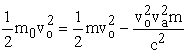Substituteand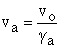Thus the Kinetic Energy for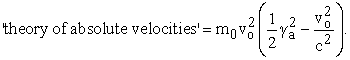(5)

If we consider ‘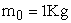’, we can tabulate the values of Kinetic energy for various values of observed velocity. Table 1 shows the results. And the Kinetic Energy is represented by Graph 1A.

This equation represents the application of ‘Double Relativity Effect’ for derivation of K.E.

But this is applicable to one film of the universe and based on special theory of Relativity. Special theory of Relativity is an ideal condition. It can not be relevant to the universe and the materialistic world what we are observing.

Observations in this universe are transformation between two consecutive films of the universe. Consciousness plays a vital role in this transformations and ‘super theory of relativity’ must be applied to the calculations of observed velocities and their kinetic energy. Thus all the observations will be influenced by ‘consciousnesses and ‘super theory of relativity’.

### 5. Observable Kinetic Energy for Experimental verification

‘Super theory of Relativity’ explains the connection of two films or frames (film can be represented as frame-explained in section.3).

In the above derivation of Kinetic Energy, the signal velocity is velocity of light ‘c’.

But in super theory of relativity the signal velocity is ‘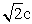So we have to replace ‘’ in place of ‘c’

Now we have to simply replace ‘’ in place of ‘c’in the Kinetic Energy equation derived above.

Then the K.E will be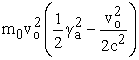(6)

Where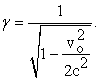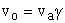Where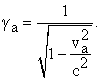The values of Kinetic Energy with increase of observed velocityare tabulated in Table 2 and represented by the Graph 2A.

This is a surprising result and shows that the Kinetic Energy will be reduced at a point with increase ofand will decrease after 4x1016. Also it will be zero at some velocity. KE is zero even if it moves with velocity means, its rest mass is becoming zero. KE is negative means, its rest mass is negative. Its rest mass is negative means, at this velocity if any particle collide with other, it will gain energy instead of releasing energy to it. The predicted values of Table 2 and Graph 2A can be observed at any ‘High Energy Physics lab’. This will be an experimental verification for ‘Concept of absolute velocities’. Thus special relativity can be modified to change the path of physics for appropriate direction.

### 6. Conclusions

1. A new version of ‘Twin Paradox’ is explained which is different from the explanation given by special theory of Relativity.

2. All the points of space in which flow of time or duration between two ticks of a clock is same called as ‘Inertial frame of Reference’.

3. The film of the universe as explained by ‘Film Theory of the Universe’ can be said as an Inertial Frame of Reference with a specific time. It is deferent from another film with another time. So all the Films of the Universe are different Inertial Frames of Reference.

4. A signal can be transferred between all the points of space of an Inertial Frame of Reference and follows special Theory of Relativity. Special Theory of Relativity is not valid for transformations from one film to another film since Inertial frame of reference is different from one film to another.

5. Since the flow of time or duration between two tics of clock is different from one film to another It is concluded as one film and is associated with absolute velocity and obeys special theory of relativity.

6. But one film of the universe has no sense in defining materialistic world. So introduction of consciousness to connect two films is mandatory and ‘Super theory of Relativity’ is implemented to describe the transformation from one film to another.

7. This interaction between one film to another has originated a new concept of ‘absolute velocities’.

8. This theory of absolute velocities clearly explained the terms ‘observer’ and ‘observation’.

9. With ‘double relativity effect’, the observed velocity has been defined, calculated and the Kinetic Energy has been derived based on the absolute velocity associated to observed velocity

As per theory of absolute velocities, Kinetic Energy of a particle of massmoving with a observed velocityis equal toWhere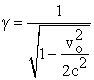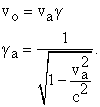10. The results have been tabulated and graphs also plotted according to the ‘theory of absolute velocities’

11. The results are different from the kinetic energy proposed by special theory of Relativity. This can be verified experimentally at any high energy lab. Its validation will substantiate the concept of consciousness in physics.

12. Thus this paper is a claim for experimental verification of Kinetic Energy in support of modification of Special Theory of Relativity as ‘theory of absolute velocities’.

### References

  Einstein,, “On the Electrodynamics of Moving Bodies”. Annalen der Physik.17: 891, 1905 (Englishversionpp.23) www.fourmilab.ch/etexts/einstein/specrel/specrel.pdf. In article View Article  Can Special Relativity Handle Acceleration? Original by Philip Gibbs 1996 http://math.ucr.edu/home/baez/physics/Relativity/SR/acceleration.html. In article View Article  Wikipedia. Twin Paradox https://en.wikipedia.org/wiki/Twin_paradox In article View Article  Kodukula, S.P. “Super Theory of Relativity-Explanation to ‘Rest Mass of Photon’, ‘Quantum Entanglement’ and ‘Consciousness’”. American Journal of Modern Physics, 3, 6, 232-239, 2014. http://article.sciencepublishinggroup.com/pdf/10.11648.j.ajmp.20140306.15.pdf. In article View Article  Kodukula, S.P. “Derivation of Siva’s Constant ‘K’ of Physics”, International Journal of Advancements in Research & Technology, 2, 1, 2013. http://www.ijoart.org/docs/Derivation-of-Sivas-Constant-K-of-Physics.pdf. In article View Article  Kodukula, S.P. New Cosmological Constants, proceedings of 9th International Symposium on Frontiers of Fundamental and Computational Physics (FFP9), Udine, Italy,7-9 January 2008. In article PubMed  PubMed  Kodukula, S.P. Double Relativity Effect & Film Theory of Universe. Lulu.com, Raleigh, North Carolina, 2009. In article  Kodukula, S.P. Heart of the God with Grand proof Equation - A classical approach to quantum theory. Lulu.com, Raleigh, North Carolina, 2009. In article View Article  Max Tegmark,“Consciousness as a State of Matter”, 2015. arXiv:1401.1219v3 [quant-ph] 18 Mar 2015. https://arxiv.org/pdf/1401.1219.pdf In article View Article  Tononi G, Koch C. “Consciousness: here, there and everywhere?” Phil. Trans. R. Soc. B 370: 20140167., 2015. In article View Article  PubMedThis work is licensed under a Creative Commons Attribution 4.0 International License. To view a copy of this license, visit http://creativecommons.org/licenses/by/4.0/